# Chart elements

Streamlit supports several different charting libraries, and our goal is to continually add support for more. Right now, the most basic library in our arsenal is Matplotlib. Then there are also interactive charting libraries like Vega Lite (2D charts) and deck.gl (maps and 3D charts). And finally we also provide a few chart types that are "native" to Streamlit, like `st.line_chart` and `st.area_chart`.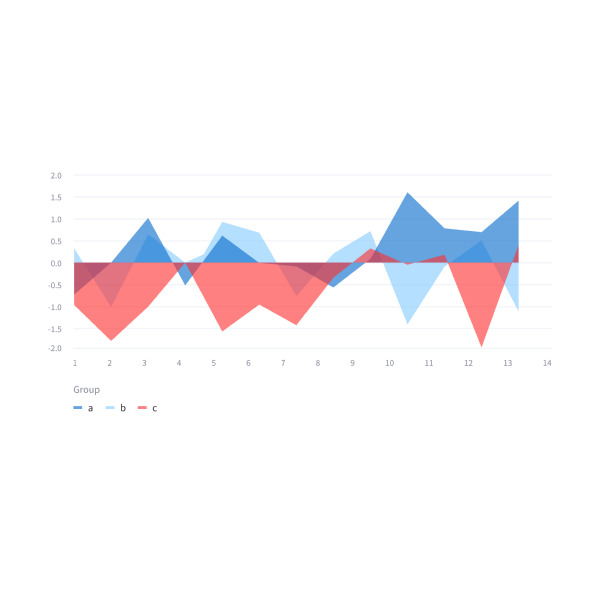#### Simple area charts

Display an area chart.

``````st.area_chart(my_data_frame)
``````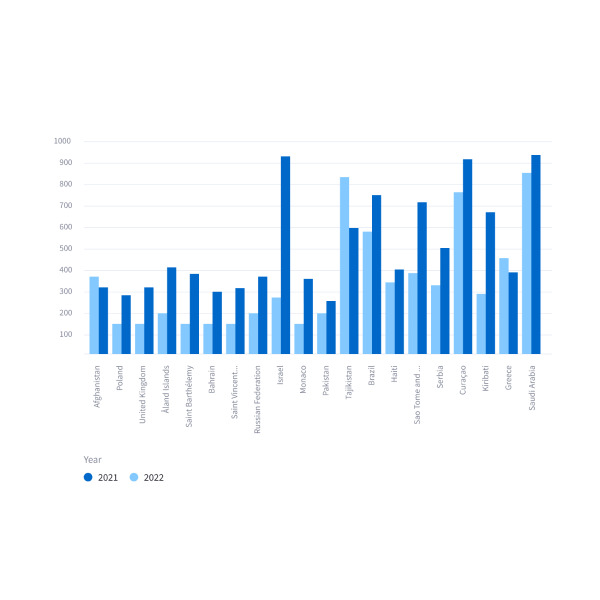#### Simple bar charts

Display a bar chart.

``````st.bar_chart(my_data_frame)
``````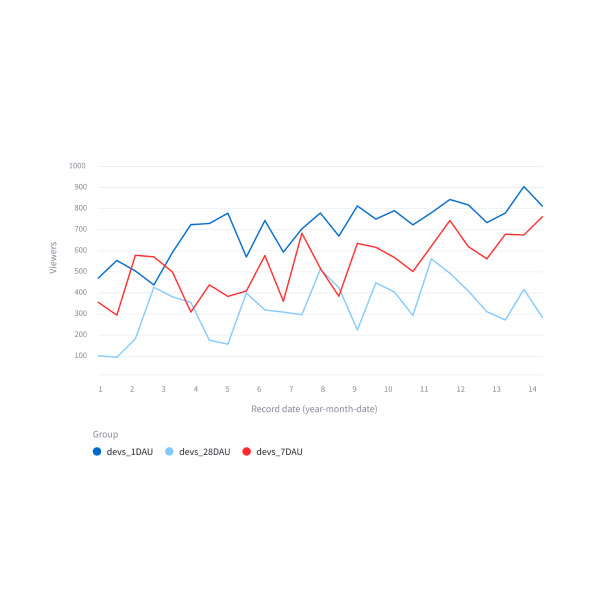#### Simple line charts

Display a line chart.

``````st.line_chart(my_data_frame)
``````#### Simple scatter charts

Display a line chart.

``````st.scatter_chart(my_data_frame)
``````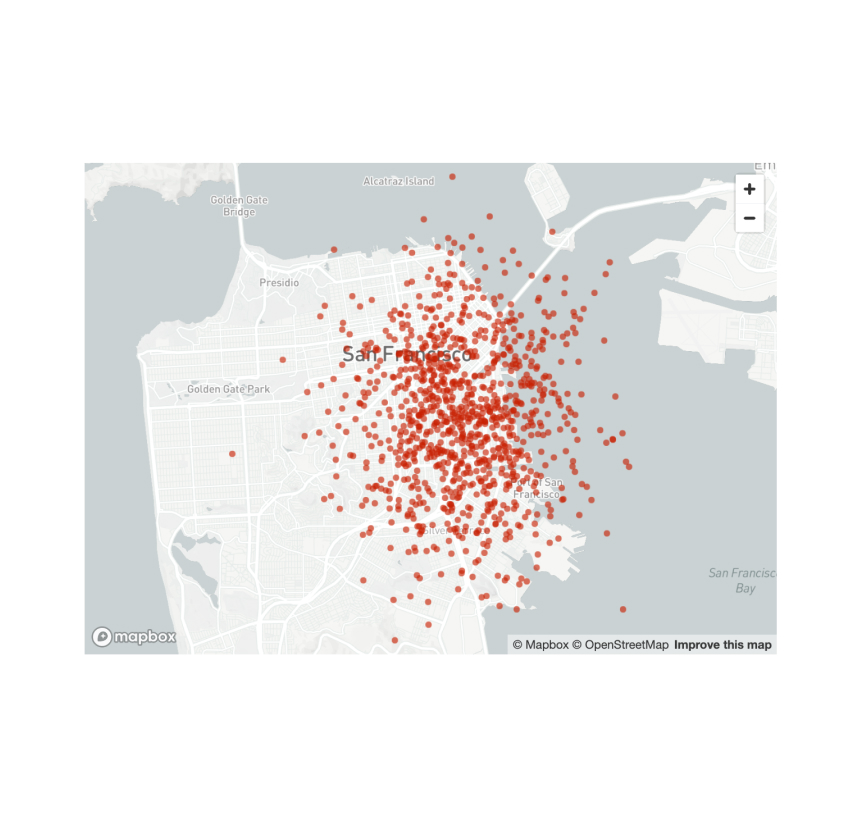#### Scatterplots on maps

Display a map with points on it.

``````st.map(my_data_frame)
``````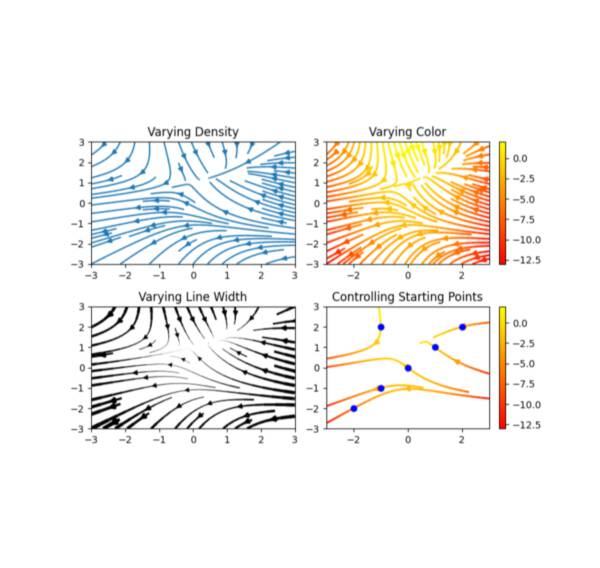#### Matplotlib

Display a matplotlib.pyplot figure.

``````st.pyplot(my_mpl_figure)
``````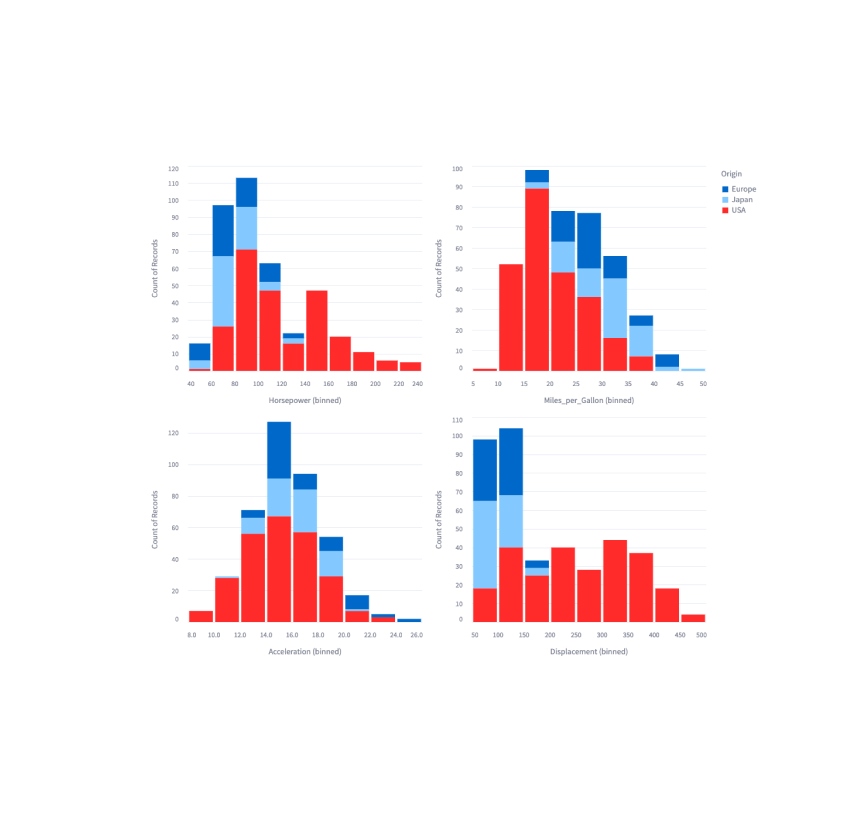#### Altair

Display a chart using the Altair library.

``````st.altair_chart(my_altair_chart)
``````#### Vega-Lite

Display a chart using the Vega-Lite library.

``````st.vega_lite_chart(my_vega_lite_chart)
``````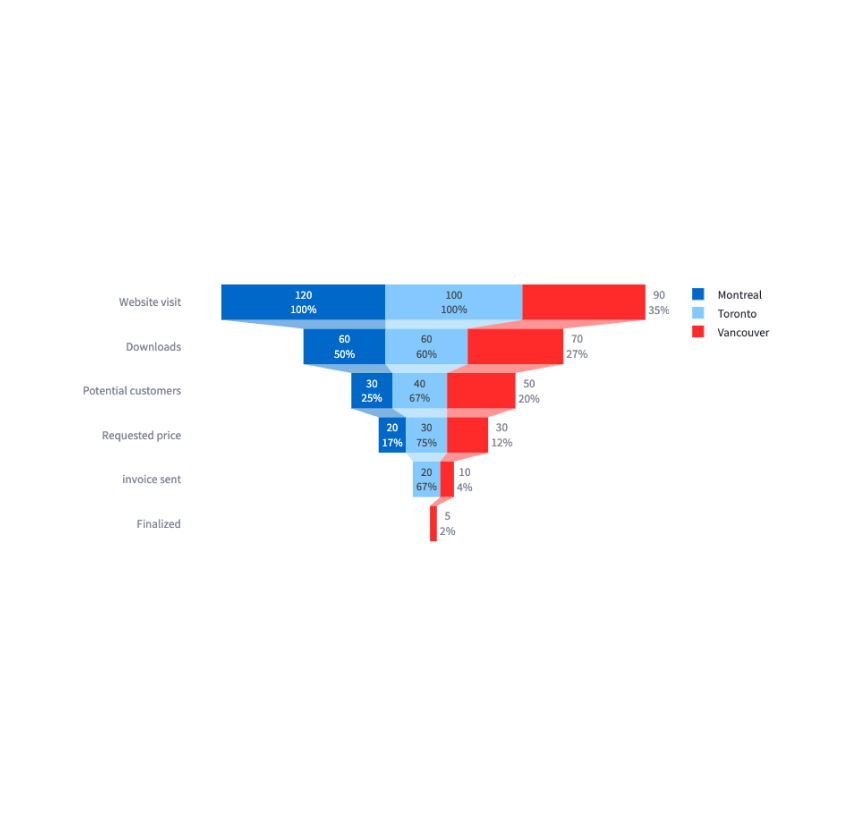#### Plotly

Display an interactive Plotly chart.

``````st.plotly_chart(my_plotly_chart)
``````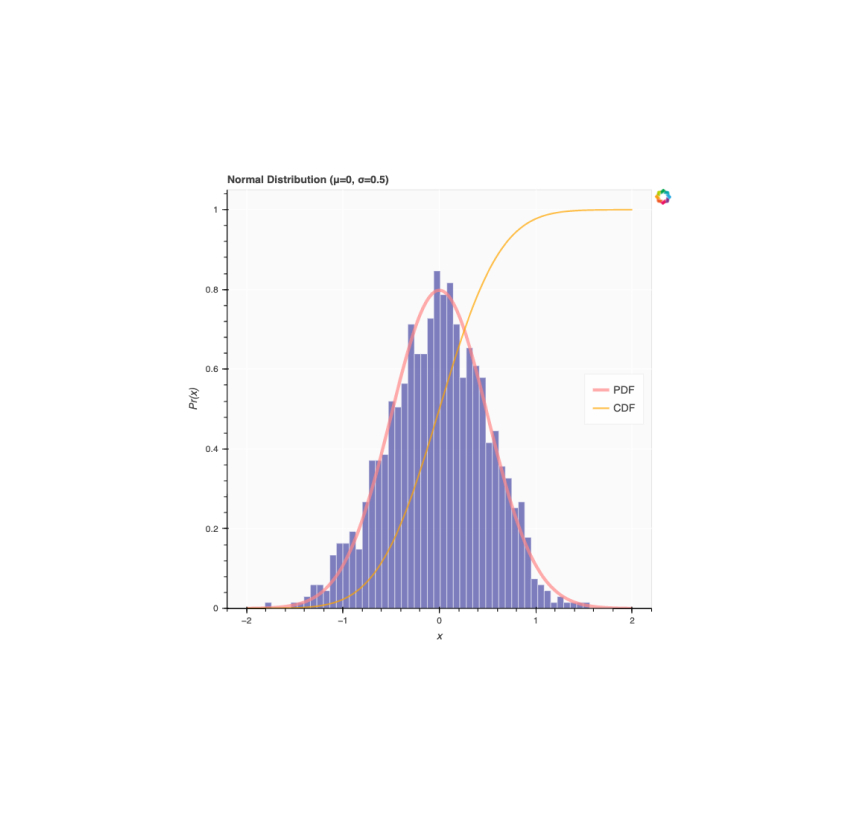#### Bokeh

Display an interactive Bokeh chart.

``````st.bokeh_chart(my_bokeh_chart)
``````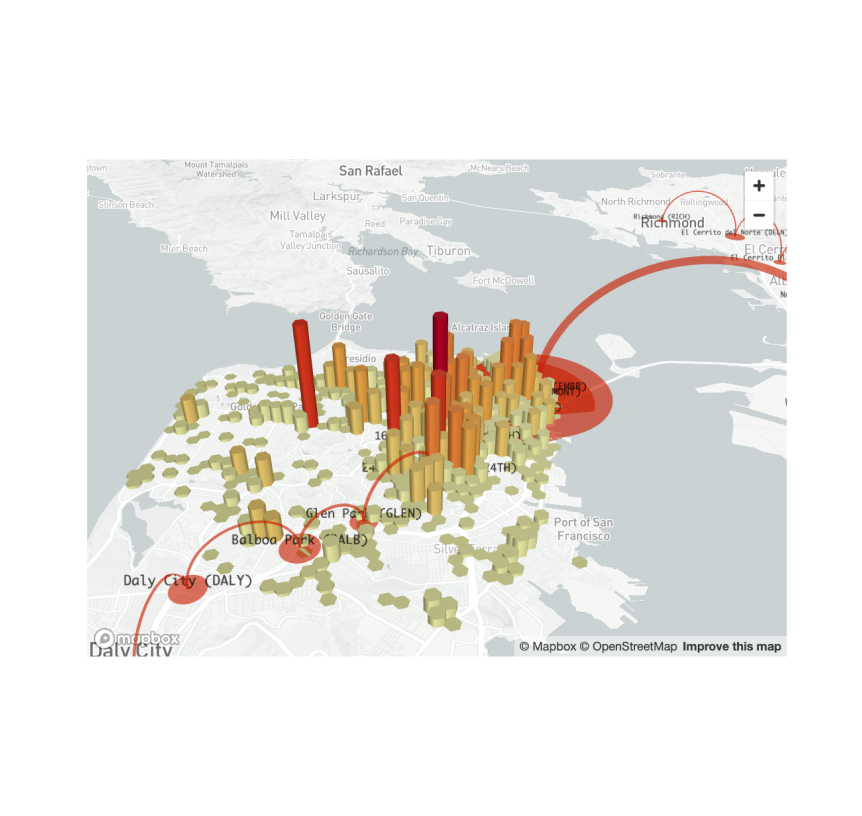#### PyDeck

Display a chart using the PyDeck library.

``````st.pydeck_chart(my_pydeck_chart)
``````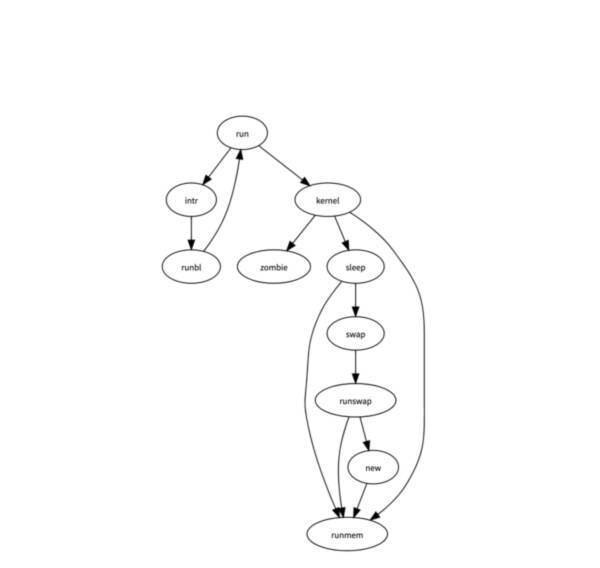#### GraphViz

Display a graph using the dagre-d3 library.

``````st.graphviz_chart(my_graphviz_spec)
``````

Third-party components

These are featured components created by our lovely community. If you don't see what you're looking for, check out our Components Hub app and Streamlit Extras for more examples and inspiration!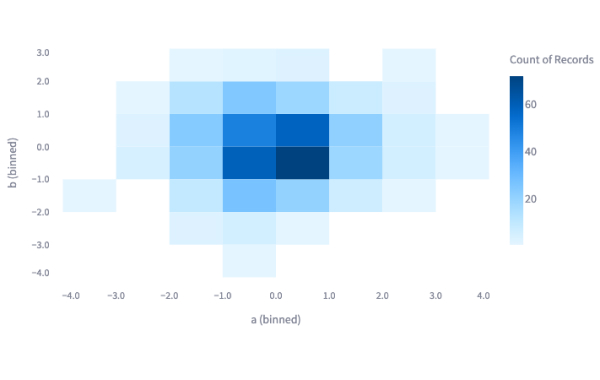#### Plost

A deceptively simple plotting library for Streamlit. Created by @tvst.

``````import plost
plost.line_chart(my_dataframe, x='time', y='stock_value', color='stock_name',)
``````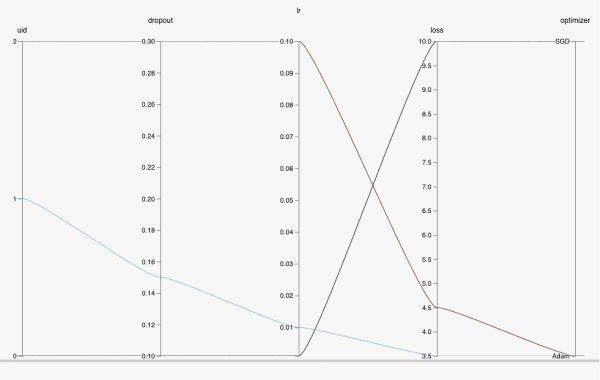#### HiPlot

High dimensional Interactive Plotting. Created by @facebookresearch.

``````data = [{'dropout':0.1, 'lr': 0.001, 'loss': 10.0, 'optimizer': 'SGD'}, {'dropout':0.15, 'lr': 0.01, 'loss': 3.5, 'optimizer': 'Adam'}, {'dropout':0.3, 'lr': 0.1, 'loss': 4.5, 'optimizer': 'Adam'}]
hip.Experiment.from_iterable(data).display()
``````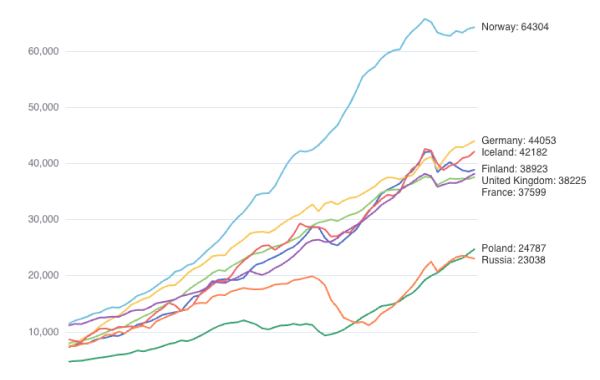#### ECharts

High dimensional Interactive Plotting. Created by @andfanilo.

``````from streamlit_echarts import st_echarts
st_echarts(options=options)
``````
forum

### Still have questions?

Our forums are full of helpful information and Streamlit experts.